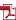# Disclosure Materials

The following are translations of IR and financial documents of Starts Proceed that were originally prepared in Japanese.

 Financial Reports Presentation Materials 28th Fiscal Period (ended October 31, 2019)FR_28th.pdfm_28th.pdf 27th Fiscal Period (ended April 30, 2019)FR_27th.pdfm_27th.pdf 26th Fiscal Period (ended October 31, 2018)FR_26th.pdfm_26th.pdf 25th Fiscal Period (ended April 30, 2018)FR_25th.pdfm_25th.pdf 24th Fiscal Period (ended October 31, 2017)FR_24th.pdfm_24th.pdf 23rd Fiscal Period (ended April 30, 2017)FR_23rd.pdfm_23rd.pdf 22nd Fiscal Period (ended October 31, 2016)FR_22nd.pdfm_22nd.pdf 21st Fiscal Period (ended April 30, 2016)FR_21st.pdfm_21st.pdf 20th Fiscal Period (ended October 31, 2015)FR_20th.pdfm_20th.pdf 19th Fiscal Period (ended April 30, 2015)FR_19th.pdfm_19th.pdf 18th Fiscal Period (ended October 31, 2014)FR_18th.pdfm_18th.pdf 17th Fiscal Period (ended April 30, 2014)FR_17th.pdfm_17th.pdf 16th Fiscal Period (ended October 31, 2013)FR_16th.pdfm_16th.pdf 15th Fiscal Period (ended April 30, 2013)FR_15th.pdfm_15th.pdf